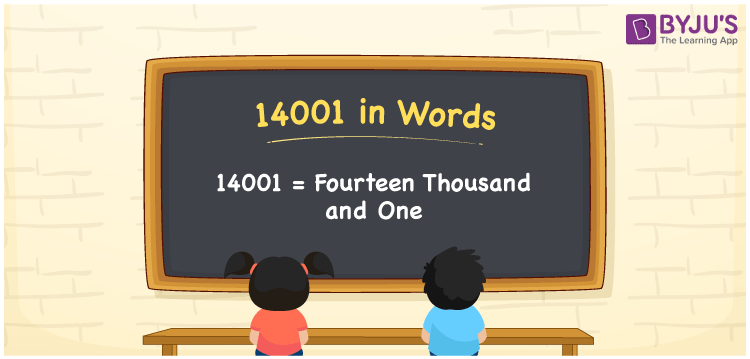# 14001 in Words

The number name of 14001 is written as “Fourteen thousand and one”. If you purchased a mobile worth Rs. 14001, it can be written as “I purchased a mobile worth Rs. Fourteen thousand and one”. For the conversion of numbers to words, we can use the place value system. In this article, let us discuss the process of writing the number 14001 in words with a complete explanation.

 14001 in Words: Fourteen Thousand and One. Fourteen Thousand and One in Numerical Form: 14001.

## 14001 in English Words## How to Write 14001 in Words?

The place values of 14001 are presented below:

 Ten-thousands Thousands Hundreds Tens Ones 1 4 0 0 1

The expanded form of 14001 is as follows:

= 1 × Ten thousand + 4 × Thousand + 0 × Hundred + 0 × Ten + 1 × One

= 1 × 10000 + 4 × 1000 + 0 × 100 + 0 × 10 + 1 × 1

= 10000 + 4000 + 1

= 14001

= Fourteen thousand and one

Hence, 14001 in words is fourteen thousand and one.

14001 in words – Fourteen thousand and one

Is 14001 an odd number? – Yes

Is 14001 an even number? – No

Is 14001 a perfect square number? – No

Is 14001 a perfect cube number? – No

Is 14001 a prime number? – No

Is 14001 a composite number? – Yes

## Frequently Asked Questions on 14001 in Words

### How to spell 14001 in English words?

14001 in words is fourteen thousand and one.

### Simplify 14000 + 1, and express it in words.

Simplifying 14000 + 1, we get 14001. Hence, 14001 in words is fourteen thousand and one.

### Is 14001 an odd number?

Yes, 14001 is an odd number.